# Fully Solved Paper Chemistry Class XII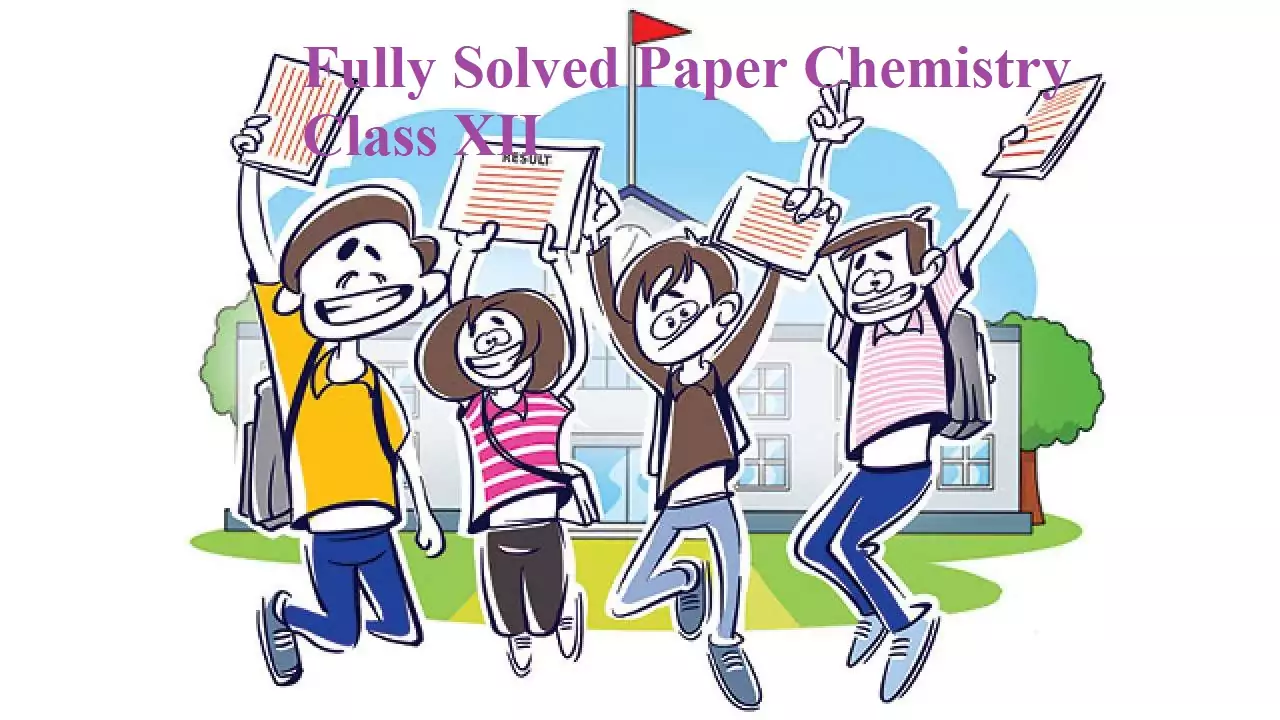Fully Solved Paper Chemistry Class XII

### M.M.70                                                                                            TIME: 3 HOURS

General Instructions:

• There are 33 questions in this question paper. All questions are compulsory.
• Section A: Q No. 1 to 16 are objective-type questions. Q.No. 1 & 2 are passage-based questions carrying 4 marks each while Q.No 3 to 16 carry 1 mark each.
• Section B: Q. No. 17 to 25 are short answer questions and carry 2 marks each.
• Section C: Q. No. 26 to 30 are short answer questions and carry 3 marks each.
• Section D: Q.No. 31 to 33 are long answer questions and carry 5 marks each.
• There is no overall choice. However, internal choices have been provided.
• Use of calculator and log table is not permitted.

Fully Solved Paper Chemistry Class XII

SECTION A (OBJECTIVE TYPE)

Alcohols, phenols, and ethers are widely used in our day-to-day life. Alcohols are classified as mono, di, tri, or polyhydric alcohols. Monohydric alcohols are further classified as primary, secondary, tertiary, allylic, vinylic, and benzylic alcohols. Alcohols can be prepared by various methods e.g. acid-catalyzed hydration of alkenes, reduction of carbonyl compounds, reduction of carboxylic acids, and the reaction of Grignard’s reagent with carbonyl compounds. On comparing the acidity of phenols and alcohols we find that phenol is more acidic because it gives H+ ion more easily and phenoxide ion gets stability due to resonance. Alcohols can be transformed into carbonyl compounds by the oxidation and dehydrogenation process. Phenols and aromatic ethers are o- & p- directing towards electrophilic substitution reactions.
The following questions are multiple-choice questions. Choose the appropriate option.
(i) With dilute HNO3 at low temperature (298K), phenol gives:
(a) O- nitrophenol
(b) 0- & p- nitro phenol
(c) m- nitrophenol
(d) picric acid
Ans (i). b

(ii) Ethanol on dehydrogenation using Cu based catalyst gives a carbonyl compound “X”. The compound “X” can also be obtained by the ozonolysis of:
(a) But-1-ene
(b) Ethene
(c) But-2-ene
(d) Propene
Ans (ii). c

OR

Correct increasing order of acidic strength is:
(a) Ethanol<Phenol<p-Cresol
(b) Phenol<Ethanol<p-Cresol
(c) p-Cresol<Phenol<p-nitro phenol
(d) p-nitro phenol<Phenol<p-cresol
Ans (ii). c

(iii)  Reaction of propanone with CH3-Mg-Br followed by hydrolysis gives:
(a) Butan-2-ol
(b) 2-Methylpropanol
(c) Propan-2-ol
(d) 2-Methyl-2-propanol
Ans (iii). d

(iv) An alcohol (in excess) when heated with conc. H2SO4 at 413K gives an ether X, which on reacting with an excess of HI gives only Ethyl iodide. The alcohol and ether would be:
(a) Ethanol & Ethoxy ethane
(b) Propanol & Ethoxy ethane
(c)Propanol & Methoxy ethane
(d) Ethanol & Methoxy ethane
Ans (iv). a

2. Read the passage given below and answer the following questions (Assertion-reason based):
Adsorption is a surface phenomenon and is of two types- Physical & chemical adsorption. On the basis of the Cause of adsorption, specificity, enthalpy of adsorption, temperature dependence, etc, physical and chemical adsorptions can be differentiated. The rate of adsorption of a gas on a solid adsorbent can be studied by plotting an isotherm called Freundlich”s adsorption isotherm. Colloids are the heterogeneous system in which one substance is dispersed in another substance. Colloids can be classified on the basis of the nature of the interaction between the dispersed phase and dispersion medium, based on physical states of the dispersed phase and dispersion medium, etc. There are so many properties of colloids that have importance like- peptization, coagulation, Tyndal effect, Brownian movement, electrophoresis, etc.
In these questions, a statement of assertion followed by a statement of reason is given. Choose the correct answer out of the following choices GIVEN.

A. Assertion & reason both statements are correct and the reason is the correct explanation of assertion.
B. Assertion & reason both are the correct statements but the reason is not the correct explanation of the assertion.
C. Assertion is a correct statement and reason is an incorrect statement.
D. Assertion is an incorrect statement and reason is a correct statement

(i) Assertion – Enthalpy of chemisorptions is high.
Reason – Chemisorption is caused by chemical bond formation.
Ans (i). a

(ii) Assertion – The gas mask is usually used for breathing in coal mines to adsorb poisonous gases.
Reason – Physisorption decreases with the increase in temperature.
Ans (ii). b

(iii) Assertion – Desorption is the process of removal of products from the catalyst’s surface.
Reason – Desorption is not essential for further adsorption.
Ans (iii). c

(iv) Assertion – The process of settling of colloidal particles is called peptization.
Reason – The coagulation power of an ion is directly proportional to its valency.
Ans (iv). d

OR

Assertion – Preferential adsorption is not required to get charge on colloidal particles.
Reason – Potential difference between the fixed layer and the diffused layer of opposite charges is called Zeta potential.
Ans. d

Following questions (No 3 to 11) are multiple-choice questions carrying 1 mark each.

3. Limiting molar conductivities of NaOH and KOH are 249.2 and 272.6 S cm2  mol-1 The difference of limiting molar conductivities of K+ & Na+ ion is
(a) 23.4 S cm2 mol-1
(b) 14.4 S cm2 mol-1
(c) 33.4 S cm2 mol-1
(d) 46.4 S cm2 mol-1
Ans 3. (a)

4. Which of the following is an example of denaturation of protein?
(a) Boiling egg

(b) Curding milk
(c) Both of these
(d) None of these
Ans 4. (b)

OR

The bond between two units of monosaccharide through the O atom is called
(b) Pyranose ring
(d) Furanose ring
Ans. (c)

5. The mixture of n-hexane and n-heptane is an example of
(a) Ideal solution
(b) non-ideal solution
(c) Dilute solution
(d) Concentrate solution
Ans 5. (a)

6. Zn is not considered as a transition element, because of
(a) Inert pair effect
(b) Filled 4s subshell
(c) filled 3d subshell
(d) Common ion effect
Ans 6. (c)

OR

Which of the following ion has electronic configuration [Ar] 3d6?
(a) Ni2+                          (b) Co2+           (c) Co3+           (d) Mn3+
Ans. (c)

7. Which of the following compound gives ethanamine in reaction with Br2 & KOH?
(a) Ethanamide                          (b) Propanamide         (c) Butanamide           (d) Ethane nitrile
Ans 7. (b)

OR

IUPAC name of the product formed when benzylamine reacts with 2 moles CH3-Cl
(a) N,N- dimethylaniline
(b) N,N-dimethylphenylmethanamine
(c) N- methyl-N phenylmethanamine
(d) N,N- dimethylbenzenamine
Ans. (b)

8. Which of the following ligands is an ambidentate ligand?
(a) OH               (b) NO2          (c) NH4+          (d) H2
Ans 8. (b)

OR

Oxidation number & coordination number of central metal atom in the complex [Co(NH3)4H2OCl]Cl2
(a) +3 & 4              (b) +3 & 6                (c) +2 & 6                 (d) +2 & 4
Ans. (b)

9. In which of the following pairs, both the ions are colored in aqueous solutions?
(a) Ni2+& Ti3+            (b) Sc3+& Ti3+           (c) Sc3+& Co2+            (d) Ni2+& Cu+
Ans 9. (a)

10. C2H5-Cl on reacting with alcoholic KOH and aqueous KOH separately gives
(a) C2H4 & C2H5OH
(b) C2H2 & C2H5OH
(c) C2H6 & C2H5OH
(d) C2H4 & C2H6
Ans 10. (a)

11. F – centers in an ionic crystal are
(a) Lattice sites containing e-                           (b) Interstitial sites containing e-
(c) Lattice sites that are vacant                        (d) interstitial sites that are vacant
Ans 11. (a)

Following questions (No 12 to 16) are Assertion- Reason type questions carrying 1 mark each.  (Instructions for these questions are as same as given in question no.2)

12. Assertion – The H- bonds are formed between a specific pair of bases in DNA.
Reason – Uracil is not present in DNA.
Ans 12. (b)

13. Assertion – Sulphur in the vapor phase show paramagnetism.
Reason – In the vapor phase, S has unpaired electrons in its antibonding molecular orbitals.
Ans 13. (a)

14. Assertion–Henry’s constant for all the gases is the same.
Reason – The mole fraction of a gas in a solution is directly proportional to the partial pressure of the gas in the vapor phase.
Ans 14. (d)

OR

Assertion – Ethanol, and water form maximum boiling azeotrope.
Reason – Mixture of ethanol and water shows a positive deviation from Raoult’s law.
Ans. (d)

15. Assertion – Carboxylic acids are more acidic than phenol.
Reason – Carboxylate ion is more stable due to delocalization of negative charge on both the oxygen atoms.
Ans 15. (a)

16. Assertion – Anisole (Methoxy benzene) gives Iodobenzene and CH3-OH on reacting with HI.
Reason
–Anisole gives o- & p- substituted product on electrophilic substitution reactions.

Ans 16. (d)

Fully Solved Paper Chemistry Class XII

SECTION B (SHORT ANSWER – I TYPE)

Q.No 17 to 25 are the short answer I type carrying 2 marks each.

17. Although chlorine is an electron-withdrawing group, yet it is o- & p- directing in electrophilic substitution reactions. Why?
Ans 17. Resonance effect predominates over –I effect of Cl. Resonating structures are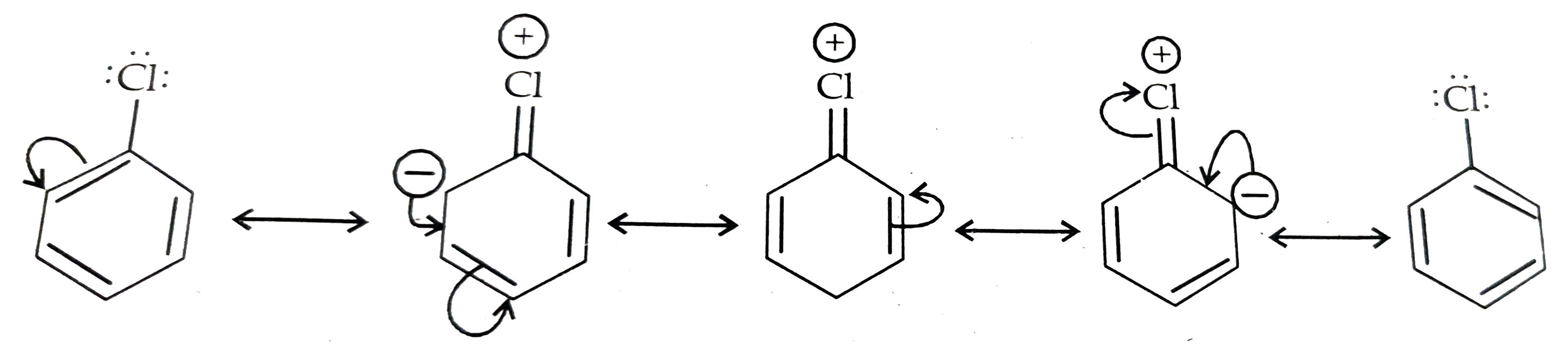OR

Carry out the following conversions (not more than two steps)
(i) Propene to propan-1-ol

(ii)Benzene to p-bromonitrobenzene
Ans:
(a) Addition of HBr in presence of peroxide and then hydrolysis with KOH (aq).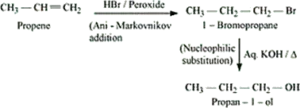(b) First bromination and then nitration of benzene.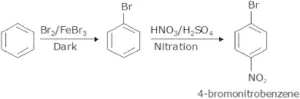18. The vapour pressure of pure benzene at a temperature is 0.850 bar. The Vapour pressure of a solution containing 0.5g of a non-volatile solid in 39g of benzene is 0.845 bar. What is the molar mass of the non-volatile solid? (Molar mass of benzene = 78g/mol).
Ans 18.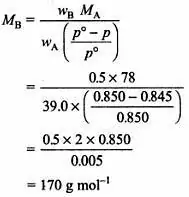19. (a) Using CFT, write the electronic configuration of iron ion in the following complexion: [Fe(CN)6]4-.
Ans 19. (a) t2g 6, eg0

(b)Write the formula of the complex – Pentaamminenitriro-N-cobalt (III).
Ans 19. (b) [Co(NH3)5(NO2)]2+

OR

(i) [NiCl4]2- & [Ni(CO)4] both have tetrahedral geometry but different magnetic behavior. Why?
Ans (i).  +2 oxidation state, 2 unpaired electrons & 0 oxidation state, no unpaired electrons.
(ii) Write the IUPAC name of [Fe(NH3)2(CN)4]
Ans (ii). Diaminetetracyanidoferrate(III)

20. A reaction is 2nd order with respect to a reactant. How is the rate affected, if the concentration of the reactant is – (i) doubled (ii) reduced to half?
Ans 20. (i) If the concentration of the reactant is doubled, i.e. [A] = 2a, then the rate of the reaction would be
R = k(2a)2
= 4ka2
= 4 R
Therefore, the rate of the reaction would increase by 4 times.

(ii) If the concentration of the reactant is reduced to half, i.e. [A] = 1/2 a, then the rate of the reaction would be
R = k(1/2a)2
= 1/4 Ka2
= 1/4 R
Therefore, the rate of the reaction would be reduced to ¼th

OR

The following results have been obtained during the kinetic study of the reaction

 A + 2B ———– →C+2D EXPERIMENT INITIAL CONC. OF [A] INITIAL CONC. OF [B] INITIAL RATE 1 0.10 0.10 3.0 X 10-4 2 0.30 0.30 9.0 X 10-4 3 0.10 0.30 3.0 X 10-4 4 0.20 0.40 6.0 X 10-4

Determine the rate law expression for this reaction.

Ans 20. Make rate law for taking all these 4 observations. Divide eq 2by 3 to get an order with respect to A and then divide eq 3 by 1 to get an order with respect to B. rate = K [A].[B]0

21. The half-life for the radioactive decay of 14C is 5730 years. An archeological artifact containing wood had only 80% of the 14C found in the living trees. Estimate the age of the sample. (Log 5 = 0.6990, log 4 = 0.6021)
Ans 21.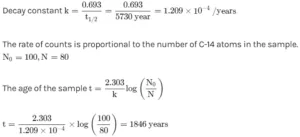22. When ethanol is treated with HBr, the following reaction takes place
C2H5-OH + HBr ————–> C2H5-Br+ H2O
Give a mechanism for this reaction.
Ans 22.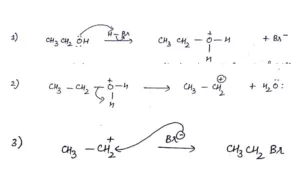23. Draw the structures of following: (a) XeF6                      (b) XeOF4

Ans 23. (a)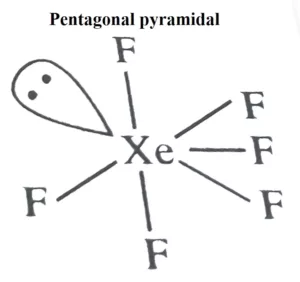(b).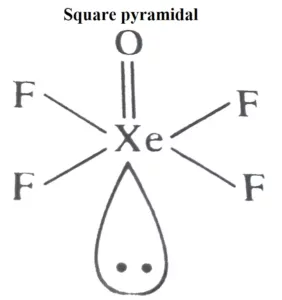24. The following haloalkanes are hydrolyzed with aq. KOH.
(a) 2- Chlorobutane
(b) 2- Chloro- 2- methylpropane
Which of the above is most likely to give a racemic mixture? Justify your answer.
Ans 24. 2-chlorobutane will give a racemic mixture as it follows the SN1 mechanism and has a chiral C.

25. Atoms of element B form hcp lattice and the atoms of element A occupy 2/3rd of the tetrahedral voids. What is the formula of the compound formed by element A & B?

Ans 25. Given that, atoms of  forms hcp lattice. So, the atoms at corners are shared by unit cells. So, its contribution is Face centered atoms contribute 1/2 and middle layer atoms contribute  each.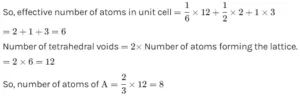Number of atoms of B = 6
A:B = A:B
8:6 = 4:3
So the formula is A4B3

Fully Solved Paper Chemistry Class XII

SECTION C (SHORT ANSWER – II TYPE)

Q.no. 26-30 are short answer II type carrying 3 marks each.

26. Give reason
(a) Zn has the lowest enthalpy of atomization in the series Sc (21) – Zn (30).
Ans (a). Weak metallic bonding.
(b) Mn can exhibit the highest number of oxidation states in the 3-d series.
Ans (b). All the 3d and 4s
(c) Cr2+ is reducing and Mn3+ is oxidizing when both are having d4
Ans (c). Cr2+ oxidizes to get t2g3 stable configuration whereas Mn3+ reduces to get d5 stable configuration.

OR

(a) E° (M2+/M) values for 3-d series elements are irregular.
Ans (a). The relative stability of M2+ and M decides E0.
(b) E° for Mn3+/Mn2+ is much more positive than E° Cr3+/Cr2+ or E° Fe3+/Fe2+.
Ans (b). Mn2+ will reduce to get d5 stable configuration.
(c) A transition metal can exhibit the highest oxidation state in its oxides and fluorides.
Ans (c). Both O & F are highly electronegative elements and they can oxidise M into higher oxidation states.

27. Arrange the following in increasing order of property specified.
(a) C2H5-NH2, (C2H5)2NH, (C2H5)3N, NH3– basic strength in the gaseous phase.
(b) C2H5-OH, C2H5-NH2, CH3-COOH – boiling point
(c) C6H5-NH2, C2H5-NH2, and C2H5-NH-C2H5 – pKb
Ans 27. (a) NH3 < C2H5-NH2 < (C2H5)2NH<(C2H5)3N > < (C2H5)3N
(b) C2H5-NH2 < C2H5-OH < CH3COOH
(c) (C2H5)2NH < C2H5-NH2 < C6H5-NH2

OR

(a) Give a chemical test to distinguish between methenamine and N-methyl methenamine.
(b) Write a chemical equation of aniline reacts with NaNO2 and HCl followed by warming with water.
(c) Aniline gives m- nitro aniline in appreciable amount along with o- & p- nitro aniline on nitration. Why?

Ans. (a) Any suitable test
(b) Phenol is formed.
(c) Due to anilinium ion which is electron-withdrawing.

28. A metal crystallizes into cubic system FCC and BCC having edge lengths 3.5A°& 3.0A° Calculate the ratio of densities of FCC & BCC unit cells.
Ans 28.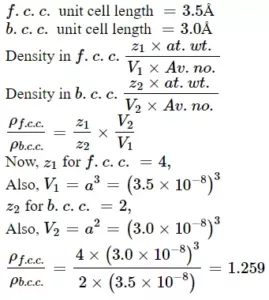29. (a) Represent Zwitterionic form of the simplest amino acid (Glycine).
(b) Why OF6 does not exist but SF6 exists
Ans 29. (a) +NH3‐CH2‐COO
(b) d-orbitals of sulphur are vacant and are available for bonding

30. (a) Arrange F2, Cl2, Br2& I2 in increasing order of bond dissociation enthalpy.
(b) N & Bi do not form pentahalides. Why?
(c) Oxygen has less negative electron gain enthalpy than S. why?
Ans 30. (a) I2<F2<Br2
(b) N does not have d orbitals and Bi has an inert pair effect.
(c) Small size and greater electron density.

Fully Solved Paper Chemistry Class XII

Q.no. 30-33 are long answer type carrying 5 marks each.

31. (a) Answer the following questions.
(i) What happens, when Cu reacts with hot & conc, HNO3?
(ii) Interhalogen compounds are more reactive than pure halogens. Why? (b)Element X has the lowest boiling point (4.2K). It is used as a diluent for O2 in the modern diving apparatus. Identify X. Which property of this element makes it usable as diluent? Why the boiling point of gas X is so low?
Ans 31. (a) (i) Cu+ 4HNO3 → Cu(NO3)2 + 2H2O + 2NO2
(ii) Due to polarity Interhalogen compounds are more reactive.
(b) “X” is helium He. He is insoluble in blood under high pressure. Very week van der Waals force as it is lightweight and monoatomic.

OR

(i) Arrange the oxoacids of chlorine in decreasing order of acidic strength.
(ii) What happens, when chlorine gas is passed through hot & conc. NaOH?
(b) A greenish-yellow gas “A” with pungent & suffocating odor is a powerful bleaching agent. “A’ on treatment with dry slaked lime gives bleaching powder. Identify “A” and explain the reason for its bleaching action. State its bleaching action is permanent or temporary.
Ans 31. (a) (i) HClO4 >HClO3 > HClO2 > HClO
(ii) 3Cl2 + 6NaOH → 5NaCl + NaClO3 + 3H2O
(b) “A” = Cl2 gas Cl2 + H2O → HCl + HOCl
HOCl → HCl + O
Coloured substance + O → Colourless substance

32. (a) An organic compound “A” (C4H8O2) was hydrolyzed with dilute H2SO4 to give a carboxylic acid“B” and an alcohol “C”. Oxidation of “C” with chromic acid gives back “B”. “C” on dehydrogenation gives ethane (C2H4). Write the chemical equations of the reactions involved.

Ans 32. (a) CH3COOC2H5 + H2O ———> CH3-COOH    + C2H5-OH
A (Ethyl ethanoate)              B (ethanoic acid)  C(ethanol)
C2H5-OH —————> CH3-COOH + H2O
C                                          B
C2H5-OH —————> C2H4 + H2O
C                              Ethene

(b) Give a chemical test to distinguish between the following pair of compounds:
(i) Propanal and Propanone
(ii) Phenol and Benzoic acid.
Ans. (b) (i) Iodoform test/ Tollen’s test or any other suitable test
(ii) Sodium bicarbonate test or neutral ferric chloride test

OR

(a) Write chemical equations involved for the following reaction
(i) Aldol condensation
(ii) Rosenmund’s reduction
Ans. (a) Aldol Condensation: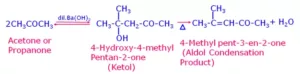(b) Rosenmunds Reaction: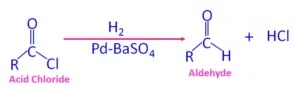(b) How will you convert?
(i) Benzyl alcohol to phenyl ethanoic acid
(ii) Propanone to propene
(iii) Ethanal to isopropyl alcohol.
(i).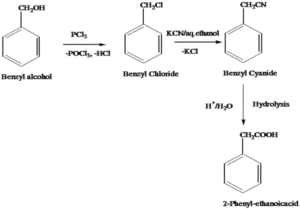(ii)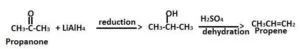33. (a) Suggest a way to determine the Ʌ0m of water.
(b) Calculate emf of the cell at 298K in which the following reaction takes place
Ni(s) + 2 Ag+(0.002M) ———–> Ni2+(0.160M) + 2 Ag(s) (Given E0 cell = 1.05 V & Log 80 = 1.9031)
Ans. (a) Ʌ0m(H2O) = [Ʌ0m(HCl) + Ʌ0m(NaOH)] – Ʌ0m(NaCl)
(b)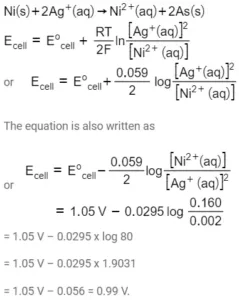OR

(a) Given the E°: K+/K = -2.93V, Ag+/Ag = 0.80V, Hg2+/Hg = 0.79V, Mg2+/Mg = -2.37V & Cr3+/Cr = -0.74V. Arrange them in increasing order of reducing power with justification.
Ans. (a) Ag < Hg < Cr < Mg

(b) Plot a graph and show the variation of Ʌm with concentration for both strong & weak electrolytes.

Fully Solved Paper Chemistry Class XII

Fully Solved Paper Chemistry Class XII